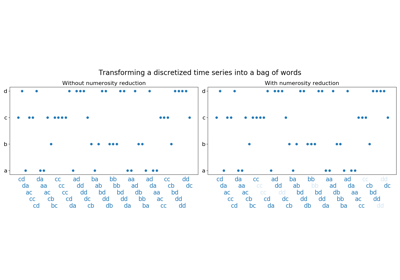# `pyts.bag_of_words`.BagOfWords¶

class `pyts.bag_of_words.``BagOfWords`(window_size=0.1, window_step=1, numerosity_reduction=True)[source]

Transform time series into bag of words.

Parameters: window_size : int or float (default = 0.1) Size of the sliding window (i.e. the size of each word). If float, it represents the percentage of the size of each time series and must be between 0 and 1. The window size will be computed as `ceil(window_size * n_timestamps)`. window_step : int or float (default = 1) Step of the sliding window. If float, it represents the percentage of the size of each time series and must be between 0 and 1. The window size will be computed as `ceil(window_step * n_timestamps)`. numerosity_reduction : bool (default = True) If True, delete sample-wise all but one occurence of back to back identical occurences of the same words.

Examples

```>>> from pyts.bag_of_words import BagOfWords
>>> X = [['a', 'a', 'b', 'a', 'b', 'b', 'b', 'b', 'a'],
...      ['a', 'b', 'c', 'c', 'c', 'c', 'a', 'a', 'c']]
>>> bow = BagOfWords(window_size=2)
>>> print(bow.transform(X))
['aa ab ba ab bb ba' 'ab bc cc ca aa ac']
>>> bow = BagOfWords(window_size=2, numerosity_reduction=False)
>>> print(bow.transform(X))
['aa ab ba ab bb bb bb ba' 'ab bc cc cc cc ca aa ac']
```

Methods

 `__init__`(self[, window_size, window_step, …]) Initialize self. `fit`(self[, X, y]) Pass. `fit_transform`(self, X[, y]) Fit to data, then transform it. `get_params`(self[, deep]) Get parameters for this estimator. `set_params`(self, \*\*params) Set the parameters of this estimator. `transform`(self, X) Transform time series into sequences of words.
`__init__`(self, window_size=0.1, window_step=1, numerosity_reduction=True)[source]

Initialize self. See help(type(self)) for accurate signature.

`fit`(self, X=None, y=None)[source]

Pass.

Parameters: X ignored y Ignored self : object
`fit_transform`(self, X, y=None, **fit_params)

Fit to data, then transform it.

Fits transformer to X and y with optional parameters fit_params and returns a transformed version of X.

Parameters: X : numpy array of shape [n_samples, n_features] Training set. y : numpy array of shape [n_samples] Target values. **fit_params : dict Additional fit parameters. X_new : numpy array of shape [n_samples, n_features_new] Transformed array.
`get_params`(self, deep=True)

Get parameters for this estimator.

Parameters: deep : bool, default=True If True, will return the parameters for this estimator and contained subobjects that are estimators. params : mapping of string to any Parameter names mapped to their values.
`set_params`(self, **params)

Set the parameters of this estimator.

The method works on simple estimators as well as on nested objects (such as pipelines). The latter have parameters of the form `<component>__<parameter>` so that it’s possible to update each component of a nested object.

Parameters: **params : dict Estimator parameters. self : object Estimator instance.
`transform`(self, X)[source]

Transform time series into sequences of words.

Parameters: X : array-like, shape = (n_samples, n_timestamps) X_new : array, shape = (n_samples,) Transformed data. Each row is a string consisting of words separated by a whitespace.

## Examples using `pyts.bag_of_words.BagOfWords`¶Bag of Words